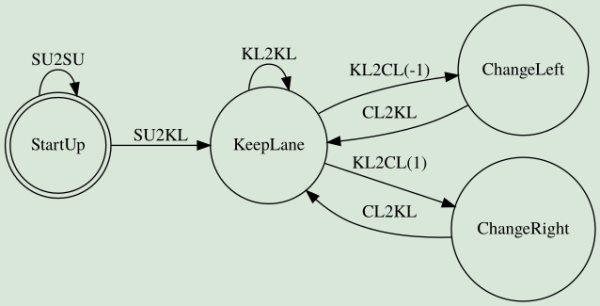## Intro

The goal of this project is to plan a path for a car to make its way through highway traffic. You have at your disposal a map consisting of a set of waypoints. The waypoints follow the center of the road, and each of them comes with the normal vector. You also know the positions and velocities of nearby vehicles. Your car needs to obey the speed limit of 50 MPH (22.35 m/s), not collide with other traffic, keep acceleration within certain limits, and minimize jerk (the time derivative of acceleration). The path that you need to compute is a set of successive Cartesian coordinates that the car will visit perfectly every 0.02 seconds.

Here's the result I got. It's not completely optimal at times, but I used large safety margins and did not spend much time tweaking it.

Path Planning

## Path planning

Udacity recommends using minimum jerk trajectories defined in the Frenet coordinate space to solve the path planning problem. This approach, however, struck me as suboptimal and hard to do well because of the distance distortions caused by the nonlinearity of the coordinate-space transforms between Cartesian and Frenet and back. Therefore, I decided to reuse the code I wrote for doing model-predictive control. However, instead of using actuations computed by the algorithm, I used the model states themselves as a source of coordinates that the vehicle should visit.

The controller follows a trajectory defined as a polynomial fitted to the waypoints representing the center of one of the lanes. In the first step, it computes 75 points starting from the current position of the car. In each successive step, it takes 25 points from the previously computed trajectory that the car still did not visit, it estimates the vehicle's state at the 25th point and uses this estimated state as an input to the solver. The points produced this way complement the path. This cycle repeats itself every 250 ms with the target speed given by:

$v = \begin{cases} v_l - 0.25 \cdot (25 - d_l) & d_l \leq 25 \\ 22.2 & d_l \gt 25 \\ \end{cases}$

Where $$v_l$$ is the speed of the leader and $$d_l$$ is the car's distance from it. Subtracting the proximity penalty tends to make speed "bounce" less than when using fractional terms. I was too lazy to tune a PID controller for this task.

## Lane selection

The most optimal lane is selected using a simple finite state machine with cost functions associated with state transitions. Every three seconds the algorithm evaluates these functions for all reachable states and chooses the cheapest move.Lane Changing FSM

The cost function for keeping the current lane (the KL2KL transition) penalizes the difference in speed between the vehicle and the leader (if any) and their proximity. The one for changing lanes (the KL2CL transition) does a similar calculation for the target lane. Additionally, it increases the cost if a follower is close enough adding a further penalty proportional to its speed.

The start up state allows the car to ramp up to the target speed before the actual lane-change logic kicks in. Doing so avoids erratic behavior arising from the penalization of speed differences.

The exact logic is here.

## Conclusions

Using the MPC approach has the advantage of letting you tune more parameters of the trajectory. You can choose how much acceleration and jerk you're going to tolerate, increasing or decreasing the perceived aggressiveness of lane changes.

I also tried incorporating the predicted trajectories of nearby vehicles into the solver's cost function, but the car ended up going out of the lane, accelerating or breaking aggressively to avoid collisions. It is something that you would do while driving in the real world, but it breaks the rules of this particular game. Another problem was that I used a Naïve Bayes Classifier to predict the behavior of other vehicles and it is not accurate enough. The mispredictions made the car behave erratically trying to avoid collisions with non-existing obstacles.

You can see the full code here.

If you like this kind of content, you can subscribe to my newsletter, follow me on Twitter, or subscribe to my RSS channel.